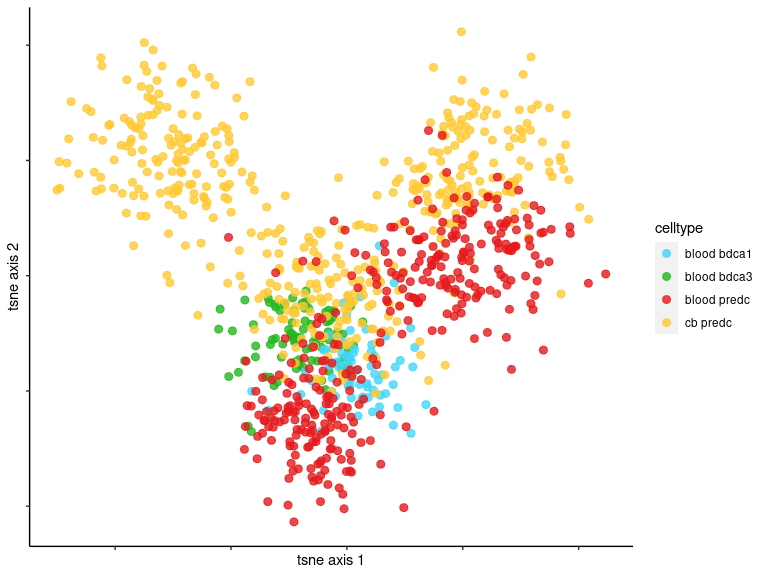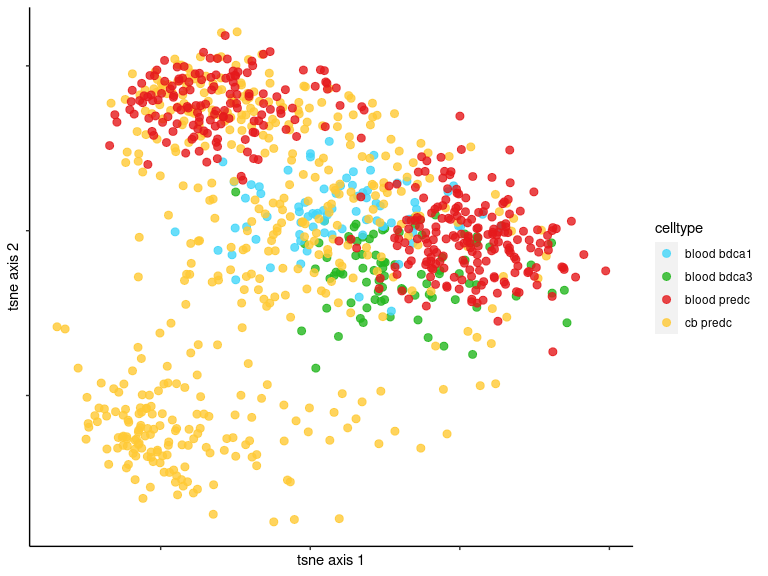Introduction

This tutorial provides an example analysis for modelling gene detection pattern as outlined in R.Li et al, 2018. The goal of this tutorial is to provide an overview of the cell type classification and visualization tasks by learning a low dimensional embedding through a class of gene detection models: that is BFA and Binary PCA.

Summary of workflow

The following workflow summarizes a typical dimensionality reduction procedure performed by BFA or Binary PCA.

1. Data processing
2. Dimensionality reduction
3. Visualization

Installation

next we can load dependent packages

Information of example dataset

The example dataset is generated from our scRNA-seq pre-DC/cDC dataset sourced from https://www.ncbi.nlm.nih.gov/geo/query/acc.cgi?acc=GSE89232 After performing all quality control procedure of genes and cells (as outlined in the paper), we then select 500 most variable genes for illustration purpose. The example dataset consists of 950 cells and 500 genes

Working with SingleCellExperiment class

The design of BFA and Binary PCA allows three kinds of input object(scData):

For illustration, here we construct a singleCellExperiment class to be the input of BFA and Binary PCA.

Gene Detection Model analysis

Binary Factor Analysis

Let $$N$$ stands for number of cells, $$G$$ stands for the number of genes, and $$K$$ stands for the number of latent dimensions.

A bfa model object computes the following parameters after fitting the gene detection matrix.

1. $$Z$$ is $$N$$ by $$K$$ embedding matrix and is named as “ZZ” in the model object
2. $$A$$ is $$G$$ by $$K$$ loading matrix and is named as “AA” in the model object
3. $$\beta$$ if there is cell-level covariates (e.g batch effect), $$\beta$$ is corresponding coefficient matrix and is named as “beta” in the model object
4. $$\gamma$$ if there is gene-level covariates (e.g QC measures), $$\gamma$$ is corresponding coefficient matrix and is named as “gamma” in the model object

We choose 3 as number of latent dimensions and project the gene detection matrix on the embedding space.

We then visualize the low dimensional embedding of BFA in tSNE space. Points are colored by their corresponding cell types.Binary PCA

We then visualize the low dimensional embedding of Binary PCA in tSNE space. Points are colored by their corresponding cell types.